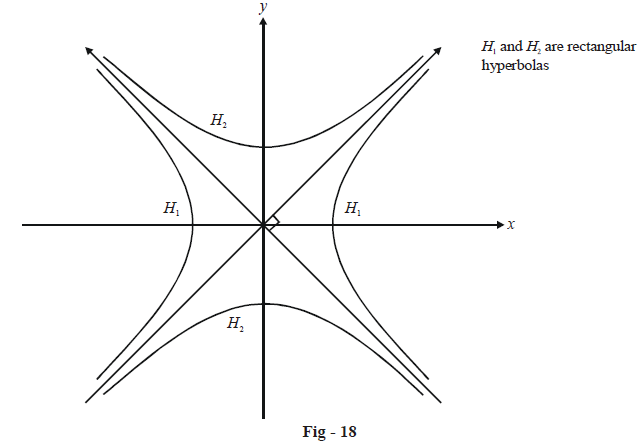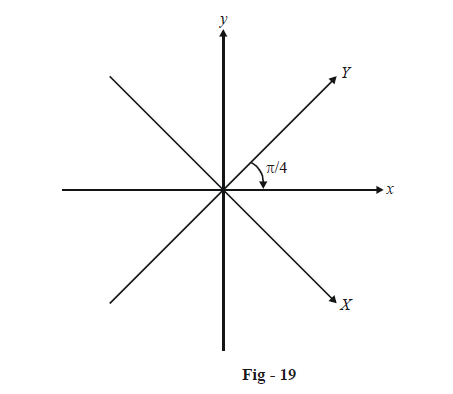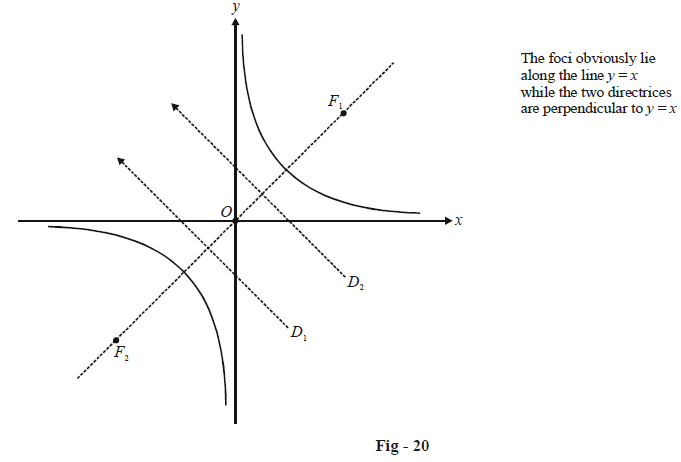# Rectangular Hyperbolas

There are more than one equivalent ways to define a rectangular hyperbola. We use any one of those.

A hyperbola is said to be rectangular if its transverse and conjugate axis are equal, i.e. if

\begin{align}&\qquad\;\; 2a = 2b \\ &\Rightarrow\quad a = b \\ \end{align}

Thus, the equation of a rectangular hyperbola is of the form

${x^2} - {y^2} = {a^2}$

The eccentricity of any rectangular hyperbola will be

$e = \sqrt {1 + \frac{{{b^2}}}{{{a^2}}}} = \sqrt {1 + 1} = \sqrt 2$

The asymptotes of a rectangular hyperbola will be

$y = \pm x \;\;\text{or}\;\; {x^2} - {y^2} = 0$

This means that the asymptotes of a rectangular hyperbola are perpendicular.

It should be obvious that the conjugate of a rectangular hyperbola  $${x^2} - {y^2} = {a^2}$$  will also be rectangular with the equation $${x^2} - {y^2} = - {a^2}.$$A lot of times, the equation of a rectangular hyperbola is written using its asymptotes as the coordinate system.

Suppose that the equation of a rectangular hyperbola is

${x^2} - {y^2} = {a^2}\,\,\,\,\,\,\,\,\,\,\,\,\,\,\,\,\,\,\,\,\,\,\,\,\,\,\,\,\,\,\,\,\, \ldots \left( 1 \right)$

and we need to rewrite this equation by using the asymptotes  $$y = \pm x$$  as our coordinate axes. Let the coordinates of any point P be  $$(x,\,y)$$ and   $$(X,\,Y)$$  in the original and the new coordinate system. We thus have, by rotation of our reference frame about the origin,

\begin{align} & x=X\cos \left( \frac{-\pi }{4} \right)-Y\sin \left( \frac{-\pi }{4} \right)=\frac{X+Y}{\sqrt{2}} \\ & y=X\sin \left( \frac{-\pi }{4} \right)+Y\cos \left( \frac{-\pi }{4} \right)=\frac{-X+Y}{\sqrt{2}} \\\end{align}Thus, (1) in the X - Y system transforms to

\begin{align}&\qquad{{\left( \frac{X+Y}{\sqrt{2}} \right)}^{2}}-{{\left( \frac{-X+Y}{\sqrt{2}} \right)}^{2}}={{a}^{2}} \\ & \Rightarrow\quad XY=\frac{{{a}^{2}}}{2} \\ & \Rightarrow\quad XY={{c}^{2}}\,\,\,\,\,\,\,\,\,\,\,\,\,\,\,\,\,\,\,\,\,\,\,\,\,\,\,\,\,\,\,\,\,\,\,\,\,\,\,\,\left( \text{where }{{c}^{2}}=\frac{{{a}^{2}}}{2} \right) \\\end{align}

This discussion shows that the equation of a rectangular hyperbola can be written in the form

$\boxed{xy = {c^2}}$

The transverse (and conjugate) axis of such a hyperbola has the length  $$2a = 2\sqrt 2 c.$$

The form of the equation for a rectangular hyperbola referred to its asymptotes could also have been obtained directly as follows : the x - axis and the y-axis are the asymptotes so the joint equation of the asymptotes is

$xy = 0.$

Since the equation of any hyperbola and that of its asymptotes differ by just a constant, the equation of the hyperbola must be

$xy = {\text{constant}}{\text{.}}$

Let us now find the foci and directrices of a rectangular hyperbola specified in the form  $$\,xy = {c^2}.$$Let 2a be the transverse axis of this hyperbola; we’ve already seen that  $$a = \sqrt 2 c.$$  Now,  $$O{F_1}$$ is simply ae i.e. $$\sqrt 2 c \times \sqrt 2 = 2c.$$

Thus,  $${F_1}$$ has the coordinates  \left(\begin{align} {2c\cos \frac{\pi }{4},\,\,2c\sin \frac{\pi }{4}}\end{align} \right) \equiv \left( {\sqrt 2 c,\,\sqrt 2 c} \right)

Similarly, $${F_2}$$  is  $$\left( { - \sqrt 2 c,\, - \sqrt 2 c} \right).$$

The directrices are at a distance   \begin{align}\frac{a}{e} = \frac{{\sqrt 2 c}}{{\sqrt 2 }} = c\end{align}  from the origin. Thus, their equations must be $$x + y = \pm \sqrt 2 c.$$

To summarize :

$xy = {c^2} \Rightarrow \left\{ \begin{gathered} {\text{Foci at }} & \left( { \pm \sqrt {\text{2}} c,\,\, \pm \sqrt 2 c} \right) \\ {\text{Eccentricity }} & \sqrt 2 \\ {\text{Transverse axis }} & {\text{2}}\sqrt {\text{2}} c \\ {\text{Directrices }} & x + y = \pm \sqrt 2 c \\ {\text{Asymptotes }} & x = 0,\,\,y = 0 \\ \end{gathered} \right\}$

The rectangular hyperbola is discussed largely using the form  $$xy = {c^2}$$  and thus it would be a good idea to be able to remember the properties of this form.

Before going on to the examples, we make the observation that any point on the hyperbola  $$xy = {c^2}$$ can be specified in terms of a parameter t as\left( {ct,\,\begin{align}\frac{c}{t}\end{align}} \right).